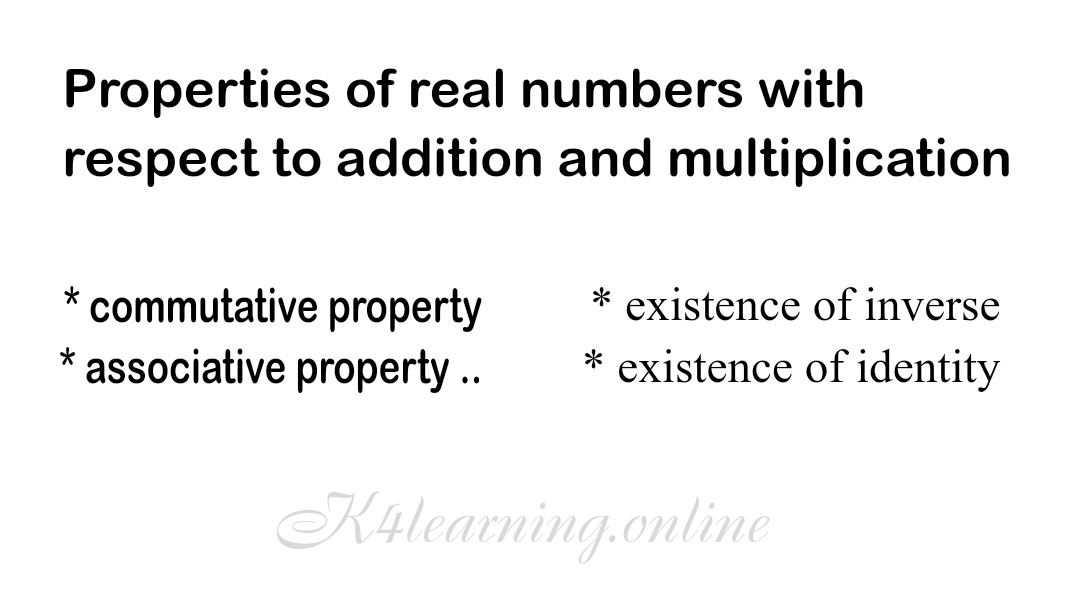# Properties of real numbers with respect to addition and multiplication

The Properties of multiplication and addition of a set R of real numbers are known as algebraic Properties of real numbers and multiplication and addition are binary operations denoted by . and +.

Binary operations on a set R of real numbers satisfy the following eight properties.Properties of real numbers with respect to addition and multiplication

## Addition Properties of real numbers

A-1

a + b = b + a

for every a and b in a set R of real numbers

A-2

a + (b + c) = (a + b) + c

for every a and b in a set R of real numbers

A-3

### Existence of a zero element (additive identity)

In a set R of real numbers there exist an element i.e

a + 0 = a & 0 + a = a

for every a in a set of real numbers

A-4

### Existence of negative elements (additive inverse)

In a set R of real numbers for every number a there exist a negative number -a i.e

(-a) + a = 0 & a + (-a) = 0

## Multiplication Properties of real numbers

M-1

### Commutative property of real numbers

In a set R of real numbers for every a and b we have

a . b = b . a

M-2

### Associative property of Multiplication

In a set R of real numbers for every a, b and c we have

a . (b . c) = (a . b) . c

M-3

### Existence of a unit Elements (multiplicative Identity)

In a set R of real numbers there exist an element 1 i.e

a . 1 = a & 1 . a = a

M-4

### Existence of resiprocal (Multiplicative inverse)

In a set R of real numbers for every number a≠0 there exist there exist a number 1/a i.e

a . 1/a = a & 1/a . a = a

There are total eight algebraic Properties in which first four are addition properties and next four are multiplication properties but there is another property of real numbers;

## Distribution property of Multiplication over addition

D-1

In a set R of real numbers for every a, b, c we have

a . (b + c) = (a . b) + (a . c)

&

D-2

(a + b) . c = (a .c) + (b . c)